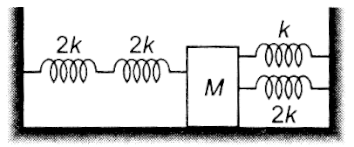Four massless springs whose force constants are 2k, 2k, k and 2k respectively are attached to a mass M kept on a frictionless plane (as shown in Fig.) If the mass M is displaced in the horizontal direction, then the frequency of the system:

# Four massless springs whose force constants are 2k, 2k, k and 2k respectively are attached to a mass M kept on a frictionless plane (as shown in Fig.) If the mass M is displaced in the horizontal direction, then the frequency of the system:1. A

$\frac{1}{2\mathrm{\pi }}\sqrt{\frac{\mathrm{k}}{4\mathrm{M}}}$

2. B

$\frac{1}{2\mathrm{\pi }}\sqrt{\frac{4\mathrm{k}}{\mathrm{M}}}$

3. C

$\frac{1}{2\mathrm{\pi }}\sqrt{\frac{\mathrm{k}}{7\mathrm{M}}}$

4. D

$\frac{1}{2\mathrm{\pi }}\sqrt{\frac{7\mathrm{k}}{\mathrm{M}}}$

Register to Get Free Mock Test and Study Material

+91

Verify OTP Code (required)

### Solution:

${\mathrm{k}}_{\mathrm{eff}}=\left[\left(2\mathrm{k}\right)\mathrm{S}\left(2\mathrm{k}\right)\right]\mathrm{P}\left[\left(\mathrm{k}\right)\mathrm{P}\left(2\mathrm{k}\right)\right]=\left[\mathrm{k}\right]\mathrm{P}\left[3\mathrm{k}\right]=4\mathrm{k}$Register to Get Free Mock Test and Study Material

+91

Verify OTP Code (required)